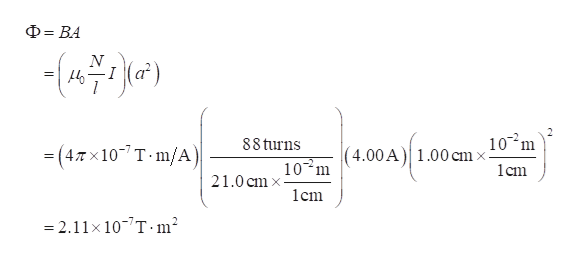# A square, single-turn wire loop ℓ = 1.00 cm on a side is placed inside a solenoid that has a circular cross section of radius r = 3.00 cm, as shown in the end view of the figure below. The solenoid is 21.0 cm long and wound with 88 turns of wire.(a) If the current in the solenoid is 4.00 A, what is the magnetic flux through the square loop in T * m^2(b) If the current in the solenoid is reduced to zero in 4.00 s, what is the magnitude of the average induced emf in the square loop?

Question
15 views

A square, single-turn wire loop ℓ = 1.00 cm on a side is placed inside a solenoid that has a circular cross section of radius r = 3.00 cm, as shown in the end view of the figure below. The solenoid is 21.0 cm long and wound with 88 turns of wire.

(a) If the current in the solenoid is 4.00 A, what is the magnetic flux through the square loop in T * m^2

(b) If the current in the solenoid is reduced to zero in 4.00 s, what is the magnitude of the average induced emf in the square loop?

check_circle

Step 1

(a) The magnetic field in the solenoid is

Step 2

The magnetic flux through the loop ishelp_outlineImage TranscriptioncloseD BA -) 10 m 4.00A 1.00cm> cm 88 turns (4Tx107 T m/A) 102m 21.0 cmx lcm -2.11x 10-7T m2 fullscreen
Step 3

(b) The current in the solenoid is reduced to zero...

### Want to see the full answer?

See Solution

#### Want to see this answer and more?

Solutions are written by subject experts who are available 24/7. Questions are typically answered within 1 hour.*

See Solution
*Response times may vary by subject and question.
Tagged in

### Physics